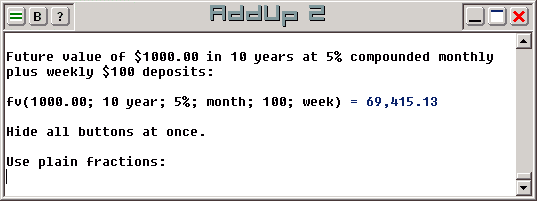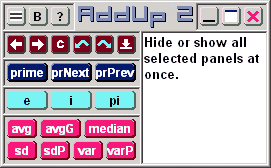# The AddUp Calculator

#### Complete.

AddUp 2 can perform conversions and calculations of all types and for all purposes: scientific, financial or computer-related.

It offers so many functions, units and scientific constants that a normal keypad could not contain enough buttons to cover them all. Instead, a variety of panels can be selected: they cover a wide range of functions you can choose from. Open the panels you require and keep the rest out of sight but still accessible as needed.

#### Flexible.

To provide flexibility, AddUp 2 uses a work area that behaves like a regular text editor. You type directly in this area to enter your calculations. Results are shown in this same area so you can use them directly for the next calculation. In fact, you don't need to use buttons at all, even though they can be convenient to have.

Nothing complicated is needed to convert measurement units: just use the "->" operator. Convert 500 grams to pounds with the expression 500 grams -> lbs. Convert nine inches to centimeters with 9 inches -> centimeters. It can hardly be simpler.#### Registeryour software

##### It's quick,affordable,fair.

Date and time operations are supported and flexible. Calculate using dates and/or times, separately or together. Convert into days or hours or years or any time unit. Add time periods to a date or subtract from a time of day. For example, calculate the number of weeks between two dates:

```([2010-01-15] - [1996-06-30]) -> weeks = 707
```

Flexibility extends to the type of numeric expressions you can enter. Use normal numbers like "12.345", scientific numbers like "1.234e+5", fractional numbers like "1 3/4", even more complicated expressions like "1 3/4 sin(30 deg)". It's all understood. You can also express values in numeric bases other than 10 simply by adding the base as a prefix. You can also use complex numbers. You can also do more!

It is not possible to fully describe on one page all the features that are available to you. But the complete software documentation is included with the product. You can read a short overview here. You can also get more details here, a page dedicated to AddUp 2.

Of course, the best way to know what AddUp 2 can do is to try it for yourself. A help file is included that contains all the documentation provided in the above links. Go ahead, download and try it!#### Clean!

AddUp contains no adware, no spyware, no virus, no malware of any kind. Certified. But beware of hacked versions distributed elsewhere. Obtain your copy directly from Orefa.com or other reputable source.

#### Get all the functionality you can expect in a calculator:

• An editable work area lets you type in your calculations directly. It is as simple as a regular text editor, and you can further process your results the same way.

• Use financial functions: future value, present value, interest rate and more.

• Exponentials functions: exp, ln, log, fact, pow, root and more.

• Fractional and whole number processing: ceil, floor, round, sRound and more.

• Random number generation, real or complex, linear or gaussian.• Seven different numeric formats for all needs: scientific (1.23e-45), currency (12,345.00), fractional (1 2/3) and more. Each one of these formats is customizable.

• Prime number functions.

• Trigonometric and Hyperbolic functions, normal and inverse.

• Statistical functions: group attributes (max, gcd, lcm and more) and distribution values (sd, var, median and more)

• Bit-level binary functions: binary-and, binary-or, binary-shift and more.

Units and conversions: convert feet to meters to feet to yards to inches to centimeters to miles...; convert grams to ounces to pounds to kilograms to pounds...; convert pints to liters to pints to quarts to gallons...; convert Celsius to Fahrenheit to Celsius to Kelvin to Rankine...; lots more!

Numeric bases: binary, octal, hexadecimal, in fact all number bases from 2 to 36 are supported in all functions and all display formats. Easily convert from any numeric base to any other.Complex numbers are natively supported and also accompanied by a specialized set of access functions.

• Annotate, edit and repeat your calculations in the work area. Save your work for later use: select either a text (.txt) or rich-edit (.rtf) document, restore it, email it, edit it with a word processor, etc.

• Comprehensive online help is included!

#### What type of calculator do you need?

 Basic Calculator Basic calculators perform general work using basic arithmetic operations. Binary Calculator A binary calculator processes numbers expressed in base-2, as series of 1s and 0s. Bit-level operations are supported as well, results being readily visible in binary notation. Complex Number Calculator Complex number calculators support complex values, numbers with a real and an imaginary components. A complex number calculator will also implement a set of specialized complex functions used to manipulate these values. Conversion Calculator Conversion calculators allow you to convert quantities expressed in one measurement unit into quantities expressed as a different unit. Date Calculator Date calculators allow you to find the difference between two dates and to either add or subtract durations from any date to identify the start and the end of a calendar period. Financial Calculator Financial calculators provide information on financial annuities such as future value, present value, interest rate, payment amounts and duration of the annuity. Hexadecimal Calculator A hexadecimal calculator processes numbers expressed in base-16. Base-16 digits are from 0 to 9 plus letters from A to F, for a total of sixteen digits. Bit-level operations are supported as well, results being easily visible in hexadecimal notation. Number Base Calculator A numeric base calculator processes numbers expressed in any number of bases besides base-10. Number bases in AddUp 2 can be any from 2 to 36. (See also binary calculator, octal calculator and hexadecimal calculator) Octal Calculator An octal calculator processes numbers expressed in base-8. Base-8 digits are from 0 to 7 only for a total of eight digits. Bit-level operations are supported as well, results being easily visible in octal notation. Prime Number Calculator Essentially, a prime number calculator can determine if a number is prime or not. It may also be able to find the next prime number after and/or before a specific value. Rational Calculator (or Fractional Calculator) A rational calculator (or a fractional calculator) processes numbers expressed as ratios, or containing a rational portion. Expressions such as 1 1/2 + 2 1/3 are understood, and the output of calculations can use the same pattern. Scientific Calculator Scientific calculators implement many mathematical, trigonometric, hyperbolic functions and specialized operators. They can also handle complex numbers. Statistical Calculator Statistical calculators process lists of numbers of arbitrary length and provide group information such as mean and median, variance and standard deviation, sum and product of all numbers. AddUp also provides attribute data on a group of numbers, such as the greatest common denominator (greatest common factor) or least common multiple. Time Calculator Time calculators allow you to find the difference between two times and to either add or subtract durations from any time to identify the start and the end of a period.